20.1
19.2
19.1
18.2
18.1
17.2
The page you are viewing does not exist in version 17.2. This link will take you to the root page.

# 3D XY Diagram

The 3D XY Diagram displays Cartesian series in a high-resolution 3D view. Refer to the 2D XY Diagram document to learn more about its 2D counterpart.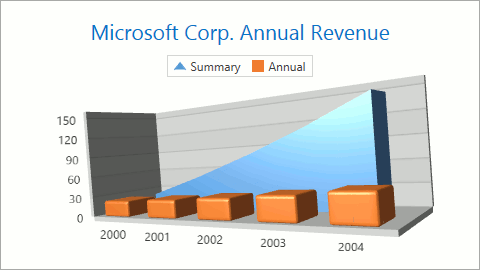This topic includes the following sections:

## Compatible Series

The diagram can only display the following compatible series:

• Area Series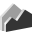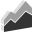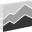• Bar Series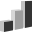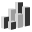• Point and Bubble Series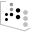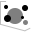## How to Create a Chart with a 3D XY Diagram

The markup below shows how to create a chart with a 3D XY Diagram:

``````<dxc:ChartControl>
<dxc:ChartControl.Titles>
<dxc:Title Content="3D XY Diagram"/>
</dxc:ChartControl.Titles>
<dxc:ChartControl.Legends>
<dxc:Legend/>
</dxc:ChartControl.Legends>
<!-- The 3D XY diagram's settings. -->
<dxc:XYDiagram3D HeightToWidthRatio="0.3"
PlaneDepthFixed="30">
<!-- The x-axis settings. -->
<dxc:XYDiagram3D.AxisX>
<dxc:AxisX3D/>
</dxc:XYDiagram3D.AxisX>
<!-- The y-axis settings. -->
<dxc:XYDiagram3D.AxisY>
<dxc:AxisY3D/>
</dxc:XYDiagram3D.AxisY>
<!-- The first Side-by-Side 3D Bar series settings. -->
<dxc:BarSideBySideSeries3D DisplayName="Series 1">
<dxc:SeriesPoint Argument="1" Value="10.5"/>
<dxc:SeriesPoint Argument="2" Value="12"/>
</dxc:BarSideBySideSeries3D>
<!-- The second Side-by-Side 3D Bar series settings. -->
<dxc:BarSideBySideSeries3D DisplayName="Series 2">
<dxc:SeriesPoint Argument="1" Value="9"/>
<dxc:SeriesPoint Argument="2" Value="11"/>
</dxc:BarSideBySideSeries3D>
</dxc:XYDiagram3D>
</dxc:ChartControl>
``````

The following table lists all the classes and properties that the markup above uses:

Class or Property Description
ChartControl The Chart Control.
ChartControlBase.Titles The Chart's title collection.
Title An individual title.
TitleBase.Content Specifies title content.
ChartControlBase.Legends The Chart's legend collection.
Legend A legend.
XYDiagram3D The 3D XY diagram.
XYDiagram3D.HeightToWidthRatio Specifies the diagram's height to width ratio as a percentage.
XYDiagram3D.PlaneDepthFixed Specifies coordinate planes' depth (thickness).
XYDiagram3D.AxisX Stores diagram x-axis settings.
AxisX3D The 3D XY diagram's x-axis.
XYDiagram3D.AxisY Stores a diagram's y-axis settings.
AxisY3D The 3D XY diagram's y-axis.
BarSideBySideSeries3D The 3D Side-by-Side Bar series.
Series.DisplayName Specifies a series's display name.
SeriesPoint A series point.
SeriesPoint.Argument The series point's argument.
SeriesPoint.Value The series point's value.

You can also access a 3D XY diagram's options at runtime as shown below:

``````// Cast Diagram to the XYDiagram3D type.
XYDiagram3D diagram = chart.Diagram as XYDiagram3D;
if (diagram != null) {
diagram.RuntimeRotation = true;
}
``````

## 3D XY Diagram's Specific Options

Below is a list of 3D XY Diagram-specific properties:

##### NOTE

Diagram pixels is a measurement unit that was introduced specially for 3D diagrams to meet their unique requirements.

The following list details specific properties for a 3D Diagram:

Zooming and Scrolling

The Diagram3D.HorizontalScrollPercent and Diagram3D.VerticalScrollPercent properties determine the distance the chart can move in relation to the central position within a diagram as a diagram width percentage. These properties can be specified using positive or negative values. If the ScrollPercent properties specify values that are too large, the series inside the diagram may be moved outside. Use the Diagram3D.RuntimeScrolling property to provide end users with scrolling capabilities at runtime.

The Diagram3D.ZoomPercent property specifies a chart magnification value. This value should be between 1 and 500 percents and is equal to 100 by default. Use the Diagram3D.RuntimeZooming property to enable zooming at runtime.

Perspective

In a perspective projection, the lines that are parallel to the object converge as they move towards a horizon line on the projection surface. The rate at which parallel lines converge is called the perspective angle. Use the Diagram3D.PerspectiveAngle property to specify a perspective angle for a 3D diagram in a perspective projection. The Perspective Angle may be equal to or more than 0, and must be less than 180.

Rotation

Use the Diagram3D.RuntimeRotation property to allow chart rotation at runtime.

3D charts can be rotated using the Ctrl+mouse pointer (design time) or mouse pointer only (runtime).

Refer to Rotation (3D Charts) for more details.